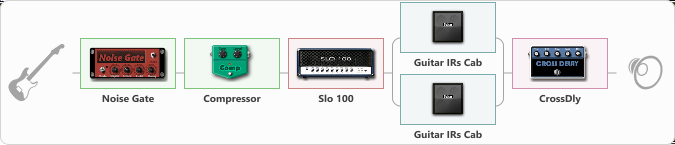# Soldano + Reverb

Discussion in 'ToneLib-GFX presets' started by rivsegura, Dec 17, 2020.

1. Soldano + Reverb

Preset name: Empty Preset

Effects chain:Effect: "Noise Gate" (Dynamics / Filter), active - "yes"
{
"Mode" = Auto
"Depth" = 50
"Threshold" = 50
"Attack" = 0
"Hold" = 5
"Decay" = 250
}

Effect: "Compressor" (Dynamics / Filter), active - "yes"
{
"Sense" = 21
"Level" = 50
}

Effect: "Slo 100" (Amp simulators), active - "yes"
{
"Gain" = 50
"Bass" = 50
"Middle" = 50
"Treble" = 50
"Presence" = 50
"Master" = 50
"Level (dB)" = 0
}

Effect: "Splitter" (Dynamics / Filter), active - "yes"
{
"A-Bypass" = Off
"A-Pan" = 0
"A-Level" = 55
"B-Bypass" = Off
"B-Pan" = 0
"B-Level" = 55

'A' branch:
{

Effect: "Guitar IRs Cab" (Cabinets), active - "yes"
{
"Model" = Bogner Ecstasy (4x12")
"Mic Position" = Middle
"Mic Distance" = Near
"Low Cut (Hz)" = 60
"Hi Cut (kHz)" = 20.0
"Mix" = 100
"Level (dB)" = 0
}
}
'B' branch:
{

Effect: "Guitar IRs Cab" (Cabinets), active - "yes"
{
"Model" = Fender Deluxe Rev (1x12")
"Mic Position" = Edge
"Mic Distance" = Middle
"Low Cut (Hz)" = 60
"Hi Cut (kHz)" = 20.0
"Mix" = 100
"Level (dB)" = 0
}
}
}

Effect: "CrossDly" (Delay), active - "yes"
{
"Time" = 756
"Feedback" = 44
"Tone" = 76
"Sens" = 0
"Mix" = 50
}

Note: You will need to download and install the ToneLib-GFX software to use the preset.

#### Attached Files:

• ###### Soldano_Reverb.tlgfx
File size:
734 bytes
Views:
2,651
NIk0lyan, Tyler Nugent and nokturnal like this.